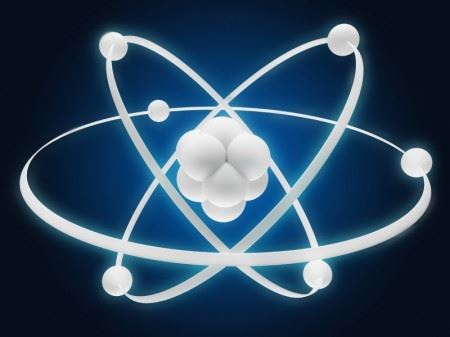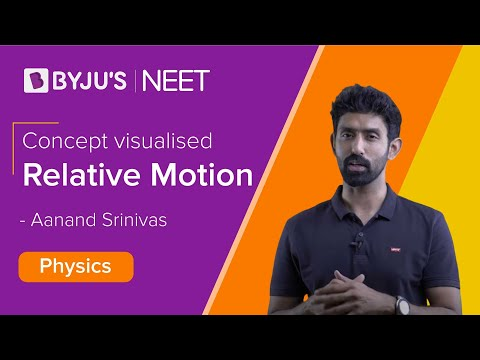Jet Set Go! All about Aeroplanes Jet Set Go! All about Aeroplanes

# Modern Physics

Modern physics often involves an advanced description of nature through new theories which are different from the classical descriptions and involve elements of quantum mechanics and Einstein’s relativity.

## What is Modern Physics?

Modern physics is a branch of physics that deals with the post-Newtonian concepts in the world of physics. It is based on the two major breakthroughs of the twentieth century: Relativity and Quantum Mechanics.
Quantum effects typically involve distances related to atoms. On the other hand, relativistic effects typically involve velocities compared to the speed of light.## Who is the Father of Modern Physics?

Albert Einstein is known as the father of modern physics. He was a 20th-century scientist, who came up with the most important ideas. One of the important works of Albert Einstein is the theory of relativity and the famous equation E=mc2.

He was awarded the Nobel Prize for physics in 1921 for his achievements in theoretical physics, particularly discovering the laws of photoelectric effect

## The video about relative motion### Modern Physics Topics

The topics which are considered as the core to the foundation of the subject are stated below:

• Atomic theory and the evolution of the atomic model in general
• Franck–Hertz experiment
• Geiger–Marsden experiment (Rutherford’s experiment)
• Gravitational lensing
• Michelson–Morley experiment
• Photoelectric effect
• Quantum thermodynamics
• Perihelion precession of Mercury
• Stern–Gerlach experiment
• Wave-particle duality
• Solid

### Modern Physics Formulas

 Quantity Formula Photoelectric effect hf=Φ+Ek Black body radiation $$\begin{array}{l}B_{v}(v,T)=\frac{2hv^{3}}{c^{2}}\frac{1}{e^{\frac{hv}{kT}}-1}\end{array}$$ Energy of photon E=hf Mass-energy E=mc2 Radioactive half-life $$\begin{array}{l}ln\frac{N_{t}}{N_{0}}=-kt\end{array}$$

Stay tuned with BYJU’S to learn more on other Physics related concepts.

## Frequently Asked Questions – FAQs

### What is modern physics?

Modern physics is a branch of physics which deals with the post-Newtonian concepts in the world of physics. It is based on the two major breakthroughs of the twentieth century: Relativity and Quantum Mechanics.

### What is the photoelectric effect?

The photoelectric effect is a phenomenon where electrons are emitted from the metal surface when the light of sufficient frequency is incident upon. The concept of the photoelectric effect was first documented in 1887 by Heinrich Hertz and later by Lenard in 1902.

### Who is the father of modern physics?

Albert Einstein is known as the father of modern physics. He was a 20th-century scientist, who came up with the most important ideas.

### What is black body radiation?

To stay in thermal equilibrium, a black body must emit radiation at the same rate as it absorbs, and so it must also be a good emitter of radiation, emitting electromagnetic waves of as many frequencies as it can absorb, i.e. all the frequencies. The radiation emitted by the blackbody is known as blackbody radiation.

### What is wien’s displacement law?

The blackbody radiation curve for different temperature peaks at a wavelength is inversely proportional to the temperature.

### What was the purpose of the Davisson-Germer Experiment?

Davisson-Germer Experiment established the wave nature of electrons.

### What is Work Function?

Work Function of metal is defined as the minimum energy required by an electron to just escape from the metal surface.

### Laws of Physics can be articulated based on _____________ according to Einstein’s Special Theory of Relativity. a) Quantum state b) Non-inertial frame of reference c) Inertial frame of reference d) None of these

Correct Option: (c)
Test your Knowledge on Modern Physics